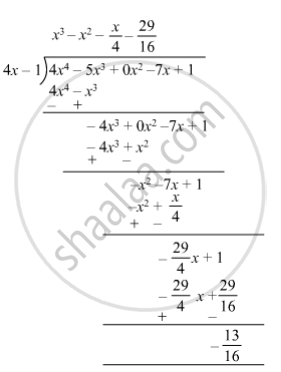SSC (English Medium) Class 8Maharashtra State Board
Share

# Divide and Write the Quotient and the Remainder. (4x4 − 5x3 − 7x + 1) ÷ (4x − 1) - SSC (English Medium) Class 8 - Mathematics

ConceptTo Divide a Polynomial by a Binomial

#### Question

Divide and write the quotient and the remainder.

(4x4 − 5x3 − 7x + 1) ÷ (4x − 1)

#### Solution

(4x4 − 5x3 − 7x + 1) ÷ (4x − 1)So, quotient = $x^3 - x^2 - \frac{x}{4} - \frac{29}{16}$ and remainder = $- \frac{13}{16}$ .

Is there an error in this question or solution?

#### APPEARS IN

Balbharati Solution for Balbharati Class 8 Mathematics (2019 to Current)
Chapter 10: Division of Polynomials
Practice Set 10.2 | Q: 7 | Page no. 66
Solution Divide and Write the Quotient and the Remainder. (4x4 − 5x3 − 7x + 1) ÷ (4x − 1) Concept: To Divide a Polynomial by a Binomial.
S